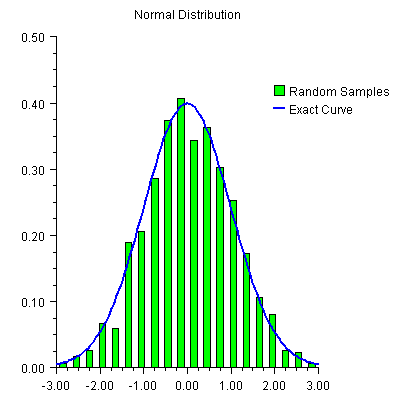JMSL Chart Programmer’s Guide
Histogram
A histogram is a bar chart in which the height of the bars is proportional to the frequencies. A histogram generally uses the same axis style as a scatter plot (i.e. the bars are numbered not labeled.)
In JMSL, histograms are drawn using the Bar class, but its setLabels method is not used.
Example
In this example normally distributed random numbers are generated and placed into 20 uniformly sized bins in the interval [-3,3]. Points outside of this interval are ignored. The bin counts are scaled by the number of samples and the bin width. The scaled bin counts are charted using Bar chart. The exact normal distribution is implemented as a ChartFunction and plotted.
The Legend is displayed by setting the legend node’s Paint attribute to true and defining the bar chart’s Title attribute. The Legend is positioned on the chart by setting its Viewport attribute.View code file

import com.imsl.math.Sfun;
import com.imsl.stat.Random;
import com.imsl.chart.*;
import java.awt.*;
import javax.swing.*;

public class SampleHistogram extends JFrameChart {

public SampleHistogram() {
int nSamples = 1000;
int nBins = 20;
// Setup the bins
double bins[] = new double[nBins];
double dx = 6.0/nBins;
double x[] = new double[nBins];
for (int k = 0; k < nBins; k++) {
x[k] = -3.0 + (k+0.5)*dx;
}
Random r = new Random(123457);
for (int k = 0; k < nSamples; k++) {
double t = r.nextNormal();
int j = (int)Math.round((t+3.0-0.5*dx)/dx);
if (j >= 0 && j < nBins) bins[j]++;
}
// Scale the bins
for (int k = 0; k < nBins; k++) {
bins[k] /= nSamples*dx;
}
// create the chart
Chart chart = getChart();
AxisXY axis = new AxisXY(chart);
chart.getChartTitle().setTitle("Normal Distribution");
chart.getLegend().setPaint(true);
chart.getLegend().setViewport(0.7, 1.0, 0.2, 0.3);
chart.getLegend().setFillOutlineType(chart.FILL_TYPE_NONE);
Bar bar = new Bar(axis, x, bins);
bar.setBarType(bar.BAR_TYPE_VERTICAL);
bar.setFillColor(Color.green);
bar.setBarWidth(0.5*dx);
bar.setTitle("Random Samples");
// plot the expected curve
ChartFunction f = new ChartFunction() {
public double f(double x) {
return Math.exp(-0.5*x*x)/Math.sqrt(2.0*Math.PI);
}
};
Data data = new Data(axis, f, -3, 3.0);
data.setLineColor(Color.blue);
data.setTitle("Exact Curve");
data.setLineWidth(2.0);
}
public static void main(String argv[]) {
new SampleHistogram().setVisible(true);
}
}